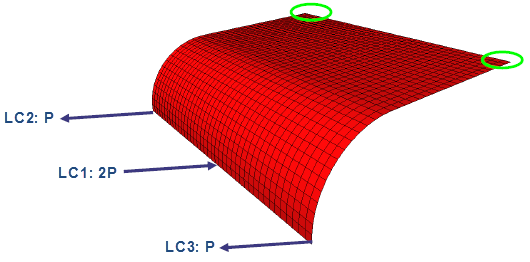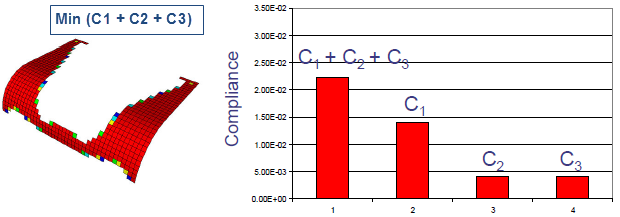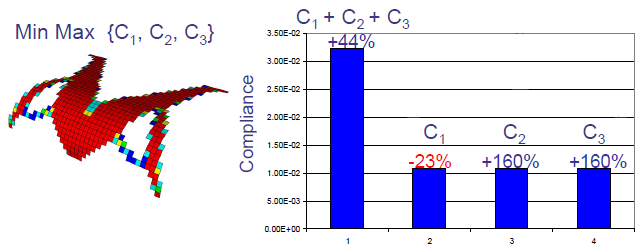Min Max Formulation for Topology

Use the Min Max formulation in a Topology study when multiple load cases act on a component.

When you subject a component to multiple load cases, the Min Max formulation for the optimization goal Best Stiffness to Weight ratio seeks to maximize the component stiffness, or minimize its compliance, for each load case independently.

For example, for a component subjected to three independent load cases, the standard optimization formulation for Best Stiffness to Weight ratio for a given mass reduction constraint seeks to minimize the total compliance. Compliance is a measure of the overall flexibility or softness of a structure, and is the reciprocal of stiffness. The total compliance is the sum of the three compliances, C1, C2, and C3, calculated independently for each load case.Shell mesh model of a plate loaded with three independent load cases: LC1, LC2, and LC3. The six degrees of freedom at the corners are fixed.Optimized shape from standard formulation. Optimization Objective Min (C1 + C2 +C3) under a 40% mass reduction constraint.
The Min Max optimization formulation seeks to minimize the maximum compliance calculated independently from the load cases and maximize the component’s stiffness for each load case. The optimized shape of the component has the maximum stiffness for each load case that can act independently.Optimized shape from Min Max formulation. Optimization Objective Min Max {C1 + C2 +C3} under a 40% mass reduction constraint. Optimized shape has equal stiffness for each independent load case.